Humanities and Social Sciences
Volume 3, Issue 5, September 2015, Pages: 249-255

The Cointegrating Relationship of Regional Growth in China

Chun-Hung A. Lin1, Chia-Ming Li2

1Department of Industrial Economics, Tamkang University, New Taipei City, Taiwan

2Division of Marketing, Delta Electronics, Inc., Taipei, Taiwan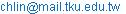(Chun-Hung A. Lin)(Chia-Ming Li)

Chun-Hung A. Lin, Chia-Ming Li. The Cointegrating Relationship of Regional Growth in China. Humanities and Social Sciences. Vol. 3, No. 5, 2015, pp. 249-255. doi: 10.11648/j.hss.20150305.23

Abstract: Since the open door policy declared in the late 1970s, the economic growth of China has been rapidly developed and accumulated. However, the widening regional economic development disparity has been brought to the concern by the government. It is doubtful that the regional economic growth would tend to be equivalent by spillover effects from some more-developed regions to other less-developed ones. The goal of this paper is to examine the long-run relationship of Chinese provincial economic performance with the consideration of the characteristic of cross-sectional dependence in the panel data covering 30 provinces in China over the period 1990-2012. We find a strong spatial dependence over in China’s regional production function. After a cointegrating relation is confirmed using the methodology of Kao (1999) and Pedroni (1999), a spatial error correction model is further applied. We find the local cointegration term is significantly negative, suggesting a long-run convergence relation for the Chinese regional economic growth.

Keywords: Regional Cointegration, Spatial Error Correction Model, Regional Covergence

1. Introduction

China’s economy has been experiencing a rapid growth rate since the economic reforms in the late 1970s that China’s GDP growth rate has maintained an annual average of 9% over the last two decades. Furthermore, recent empirical literatures on economic growth have also found that at cross-regional level the income disparity in China has been increasing across regions over the recent years (Xu and Zou, 2000; Maasoumi and Wang, 2008; Zou et al., 2008 Zhou, et al., 2014; Liao and Wei, 2015). The widening regional economic disparity has brought about an important issue in Chinese economic development. It shows the evidence of a significant cross-sectional dependence in the GDP distribution, which displays a clear spatial-clustered effect in the coastal provinces. LeSage (1999) suggests that observations associated with spatial units such as zip-codes, counties, states, census tracts and so on, should consider spatial correlated, or might reflect measurement errors.

Due to the shortcoming of the conventional tests of spatial dependence, which is usually exogenously given a connection or spatial matrix for regional relevance, this paper applies the test for spatial correlation in residuals of the estimated model and adopts the test for cross-sectional dependence (CD test) developed by Pesaran (2004), which allows the panel data with a short time span T and a large number of cross sections N . Since our panel data consist of 30 provinces of China for the periods 1990 to 2007, it is suitable for the assumption of Pesaran (2004).

As mentioned above, the spatial correlation effect in panels should be considered. Indeed, the panel data might be nonstationary by allowing for a feedback effect from the current dependent variable to the future explanatory variables. However, the spatial econometric researches using panel data usually ignore the issue of non-stationarity by assuming panel data are the temporally static (Elhorst, 2003; 2009). Moreover, a general panel data model also usually assumes the spatial independence. In this paper we attempt to integrate the issue of space and time in panel data and argue that the spatial variables are cointegrated in the long-run. Beenstock and Felsenstein (2010) propose the method of spatial vector error correction model (SpVECM) to overcome these problems. The SpVECM concurrently contains both the temporal dynamics and spatial dynamics by including the local cointegration and spatial cointegration in the estimated model. Local cointegration indicates that the nonstationary panel data are cointegrated "within" spatial units but not "between" them, while spatial cointegration indicates the non-stationary variables are cointegrated "between" spatial units but not "within" them (Beenstock and Felsenstein, 2010; Baker, Merkert and Kamruzzaman, 2015).

The purpose of this paper seeks to apply the panel data with the characteristic of cross-sectional dependence and examine the long-run relationship of Chinese provincial economic performance. Using an annual panel dataset for 30 provinces in China over the period 1990-2007, we provide the procedure of statistical and systematical tests for the panel data with the characteristic of cross-sectional dependence and apply the spatial error correction model provided by Beenstock and Felsenstein (2010) to measure the long-run effect on provincial GDP growth in China.

2. Methodology and Data

2.1. Determinants of Regional Production Function

In order to examine the long-run impact on regional comparisons of Chinese economic performance, we apply a dynamic spatial panel production function, which is augmented from the growth model of Solow (1957) and contemporaneously consider the feature of human capital (Mankiw et al., 1992). An augmented Cobb-Douglas production function for Chinese provincial output is employed in this study as follows.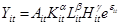(1)

where Y is provincial output, K is physical capital stock, L is labor force, and H is human capital. The subscripts i and t refer to the province i and the current year t. In general, we anticipate that the impacts of physical capital stock, labor force, and human capital on provincial output are positive. A is the technical factor. Based on the previous related studies, we know that the technological channels come from two sources: (1) inward FDI and international technology import; (2) internal leading research and development (R&D) activities. The international technology sources indicate that multinational enterprises (MNCs) usually have advantaged technologies, which can spill over to local firms through technological externalities. Moreover, because the in-house R&D activities were not quite spirited in the early 1990s, China’s spectacular economic growth is believed to be largely contributed by the implementation of its "open door policy" in the late 1970s. It implies the foreign-owned enterprises play a more important role for China’s economic growth relative to the in-house R&D. Therefore, we emphasize more on the role of FDI to determine its influence on regional economic performance. We assume the technical factor A is associated with the provincial inward FDI. Finally, e denotes the error term which reflects the effects of unknown factors. We rewrite equation (1) as a log-linear form of the augmented Cobb-Douglas production,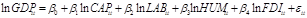(2)

where ln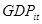is the logarithm of regional gross domestic product (GDP) in region i at year t. CAP and LAB are the two core physical inputs that denote fixed capital stock and labor force, respectively. We follow Yao (2006)'s setting of perpetual inventory method for fixed capital stock, assuming that the depreciation rate is 7.5% and the growth rate of capital is 15%, which imply the average life of capital equipment is 13.3 years. Labor force is measured as the labor population who are older than 15 years old in region i and at year t. HUM is measured as the number of students per 10,000 population enrolled in the higher education level. The error term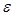has the characteristic of heterogeneity, which is assumed to include a common regional factor that does not vary over time. That is,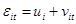, where u is a region-specific effect that varies across regions but is fixed within a region over time, and v is a "white noise" error term.

2.2. Testing for Cross-Sectional Dependence in Panel Data

The conventional tests of spatial dependence are usually exogenously given a connection or spatial matrix for regional relevance. But the terminal inference and analysis of the spatial dependence test could critically depend on the choice of the spatial matrix. We adopt a cross-sectional dependence (CD) test in panel data proposed by Pesaran (2004). The test statistic is described as below.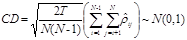(3)

where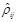is the sample estimate of the pair-wise correlation of the residuals,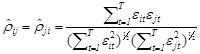, whereare the residual which obtained from the equation (2). The null hypothesis of CD test assumes no cross-sectional or spatial dependence among the sample. The CD test can provide sufficient evidence whether the cross-sectional correlations are present between pairs of regions for all variables. Using the data we collect for the paper, the statistics of CD test is 53.23 (p-value=0.000) in the fixed effect panel model of equation (2) and 56.16 (p-value=0.000) in random effect panel model of equation (2), respectively. The results strongly indicate the existence of cross-sectional dependence in Chinese provincial production function model.

2.3. Testing for Stationarity in Panel Data

Pesaran (2007) proposes an individual cross-sectional augmented Dickey-Fuller (CADF) test to examine stationary of variables in panel data, which modifies the usual Im, Pesaran and Shin (2003)’s unit root test (IPS) with the lagged cross-sectional mean and its first difference to capture the cross-sectional dependence arising through a single factor model. The CADF test is described as follows.(4)

where, which is the average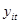of all provincial observations at time t. p is the lagged order of the model. The null hypothesis of the test can be expressed as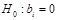for all i against the alternative hypothesis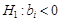for some i. We donate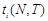as the t-statistic of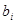in the CADF regression. The t-statistic is used to construct a cross-sectionally augmented version of the IPS test: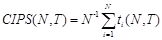(5)

where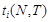is the cross-sectional augmented Dickey-Fuller statistics for the province i. In addition, to avoid the problem of an extreme statistic caused by a small sample, Pesaran (2007) constructs a truncated version of the CIPS test, which is denoted as a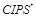test.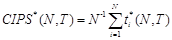(6)

where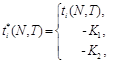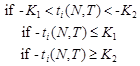The parameters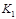and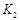are positive constants based on the simulated values of CADF. For models with an intercept and trend, Pesaran (2007) suggests using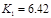and.

2.4. Testing for Panel Cointegration

To avoid the problem of spurious regression, we must investigate the cointegration correlation of variables. If y and x are spatially panel unit root variables with the same order of integration, SI(1), in general any linear combination of y and x is also SI(1). If a linear combination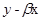is stationary, it implies the linear combination of x and y has a cointegrated relationship.

Kao (1999) and Pedroni (1999), which are two well-known cointegration tests, propose residual-based cointegration tests. Kao (1999) develops the DF and ADF-type unit root tests for the error term of the panel regression model as a test for the null of no cointegration. There are four DF-type tests,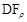,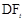,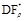and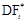, while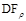and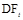are based on the strong exogeneity of the regressors and errors,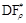and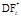are for the cointegration with endogenous relationship between regressors and errors. The null hypotheses of Kao (1999)’s cointegration tests assume a cointegration in a panel data model.

Pedroni (1999, 2004) proposes several tests for the null hypothesis of no cointegration in a panel data model, allowing for considerable heterogeneity. Seven tests follow asymptotically a standard normal distribution after a suitable normalization. Among them, four of these statistics, called panel cointegration statistics, are within-dimension based statistics. The other three statistics, called group mean panel cointegration statistics, are between-dimension panel statistics. The former statistics pool the autoregressive coefficients across different members for the unit root tests on the estimated residuals, while the later are based on estimators that simply average the individually estimated coefficients for each member i. The null hypothesis of no cointegration is tested (, where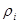is the autoregressive coefficient of estimated residuals,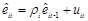). The alternative hypothesis of within-dimension cointegration statistics is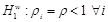, while the alternative hypothesis of between-dimension cointegration statistics is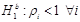.

2.5. The Estimation of Regional Production Function

Assume that y and X are the variables with a spatial panel unit root (non-stationary), and therefore the combination of y and X could be cointegrated. Moreover, according to the result of CD test, we evidence the existence the cross-sectional correlation in equation (2). We can rewrite equation (2) and present the regional production function with spatial lag terms as below.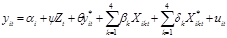(7)

where i denotes the cross-sectional dimension, t denotes the time-series dimension.is the logarithm of provincial GDP. Z is a vector of observed common factors that are assumed to affect all spatial units. X denotes a matrix of other explanatory variables, presented as the logarithm type, including physical capital stock (CAP), labor force (LAB), human capital (HUM), and foreign direct investment (FDI). The superscript asterisked variables refer to spatial lags terms which are calculated by the sum of spatially weighted values of neighboring locations. They are defined as below.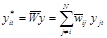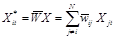(8)

where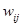is the spatial weight,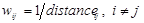;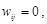i=j. We use the longitude and latitude of provincial capital cities to calculate the straight distance from region i to region j. The spatial weight matrix can be defined as below.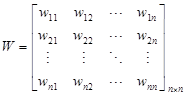(9)

The spatial weight matrix intends to capture the spatial spillover effect of other neighboring provinces and gives each spatial unit the relative weight. However, to ensure the sum of weight in each row equal to one, we adjust the spatial weight matrix to a row-standardized matrix. The row-standardized spatial weight matrix is presented as follows.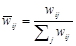,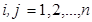.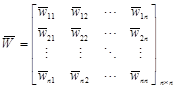(10)

In equation (3), as the variables are cointegrated, implies that at least exists an one-way error correction in the spatial panel error correction model (SpECM). We follow the setting of Beenstock and Felsenstein (2010)’s SpECM. The estimation equations are described as below.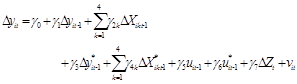(11)

where u and u* are derived from the estimated residuals of equation (3) (u*=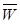u).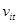is the residual of SpECM. We denote u is the "local error correction" term, while u* is the "spatial error correction" term. Using the SpECM, we can find the dynamic long-run equilibrium in the model and carry out the causal relationship tests.

When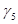is non-zero, there is local error correction; and when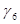is non-zero, there is spatial error correction. When bothandare non-zero, i.e. both types of error correction occur, we refer to this as "global error correction". In equation (11),is expected to be negative since yit is expected to decrease as it corrects itself toward to its equilibrium value (i.e. so-called convergence), whileis expected to be positive since a positive neighboring spillover is expected to increase the local GDP.

The data used in this study contain 30 provinces in China for 23 consecutive years, 1990-2012, yielding 690 observations. The period is sampled because China’s economic activity has risen significantly since the early 1990s. We ignore the Tibet autonomous province from our dataset because Tibet lacks for several data in control variables. Therefore, the dataset used in exploring the long-run relationship among the regional production function with cross-sectional dependence can provide valuable insights for Chinese provincial production function cointegrated relation. Table 1 shows the the definitions of the variables used in our analysis and the basic statistic description.

Table 1. Variable Definitions and Basic Descriptive Statistics.

 Variables Definition Mean (Std. Dev) GDP Gross domestic product For i province (millions RMB) 344,871.7 (447,108.5) CAP Capital stock: it is calculated using the perpetual inventory method (millions RMB) 1.63e+07 (1.25e+08) LAB Labor population who are older than 15 years old (10,000 persons) 4,937.283 (9,304.88) HUM Human capital: Number of students enrolled in high education per 10,000 population (persons). 74.44084 (88.887) FDI Foreign direct investment inflow (RMB million), adjusted by annual average exchange rate. 145,768.3 (274,608)

Notes: The means and standard errors are calculated by pooling data for the 1990-2012 period. All monetary figures are at 2002 prices.

3. Empirical Results

In the preliminary step we apply the panel unit root test provided by Pesaran (2007), which allows cross-sectional dependence in the panel data model. The model used to test the unit root hypothesis is the one with intercept and trend. We set the lag lengths order at the maximum equal to 2 (p=0, 1, 2). The results indicate all of the variables exhibit non-stationarity in both CIPS and CIPS* statistics. We further take the first differencing for the series of these variables and the results show they become stationary, implying that these variables could be integrated of order one. Given that regional GDP and the inputs of regional GDP have a panel unit root, we further examine two types of panel cointegration tests developed by Kao (1999) and Pedroni (1999, 2004) to evidence the existence of a long-run relationship among the variables included in the regional production function model. The results of panel cointegration tests suggest the rejection of null hypothesis of no cointegration among the variables in the regional production function at the 5% statistical level except Kao (1999)’sandstatistics. The estimated results imply that the variables are clearly cointegrated and hence exists an error correction relationship, suggesting that an error correction model is necessary to be used in the estimation.

To obtain a comparison base, we report the ordinary least square (OLS) estimation results for the benchmark model, which are presented in Table 2. The empirical results obtained by using fixed effect (FE) model are reported in Models 1 and 2. We perform the cross-sectional dependence (CD) test provided by Pesaran (2007), AR(1) test, and also adopt Breusch and Pagan (1979)'s Lagrangian multiplier (LM) test for random effects to test the heteroskedasticity in error terms. Furthermore, in order to check the robustness of estimated results, we adopt the feasible generalized least square (FGLS) estimation in Model 3, which is to capture the heteroskedasticity with cross-sectional correlation and common AR(1) coefficient for all panels.

Table 2. The benchmark models of regional production function.

 Variables Model 1 (FE) Coeff. (Std. err.) Model 2 (FE) Coeff. (Std. err.) Model 3 (FGLS) Coeff. (Std. err.) CAP 0.2398*** (0.0199) 0.0059 (0.0055) 0.0339*** (0.0053) LAB 0.0042 (0.0316) 0.0721** (0.0337) 0.9303*** (0.0048) HUM 0.5348*** (0.0301) -0.0146 (0.0301) 0.4398*** (0.0044) FDI 0.2069*** (0.0142) 0.0275*** (0.0041) 0.0130*** (0.0005) GDP* 1.0029*** (0.0317) 1.0782*** (0.0113) CAP* -0.0169 (0.0261) -0.2498*** (0.0077) LAB* -0.0701 (0.0341) -0.9321*** (0.0054) HUM* 0.0061 (0.0320) -0.4053*** (0.0075) FDI* -0.0207 (0.0105) 0.0951*** (0.0041) intercept 4.7354*** (0.2617) 0.0541 (0.1108) 0.6142*** (0.0446) R2 0.893 0.994 0.902 CD test 53.225*** -2.575 AR(1) test 982.17*** 1194.89*** B-P LM testa 608.45*** 2849.24*** Hausman test 599.77*** 3735.94*** # of obs. 630 630 630

Notes: All variables are expressed in logarithms. *, **, and *** indicate the parameters that are significance at 10%, 5%, and 1% probability level respectively.

In Model 1, the presences of CAP, HUM, and FDI are found to have significantly positive effects at 1% level, while variable LAB is not significant. However, the cross-sectional dependence test shows a significant existence of cross-sectional dependence in Chinese provincial production function model. Therefore, validating the problem of spatial unit correlation effects should be considered. We add the spatially lagged dependent variable,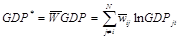, and the spatially lagged terms of other explanatory variables into the equation (2) to capture the spatial correlation effects. In Model 2, the result of CD test shows no rejection of the null hypothesis of no cross-sectional dependence, implying the spatial correlation effects on regional production function have been controlled in the estimation of Model 2. The estimated elasticity of GDP* in Model 2 is positive and significant, indicating that it will spill over to the local region if the neighboring provincial GDP increases.

In Models 1 and 2, we also test the autoregressive coefficient of estimated residuals, AR(1), and the Lagrangian multiplier (LM) test for random effects developed by Breusch and Pagan (1979). The estimated results indicate the existence of heteroskedasticity with cross-sectional correlation and AR(1) problem in panels. Therefore, we adopt the generalized least square estimation to control the problems of heteroskedasticity and AR(1) in Model 3.

In Model 3, the estimated results show the variables CAP, LAB, and HUM are significantly positive, and the variables CAP*, LAB*, and HUM*are significantly negative effects at 1% statistic level. We consider that the impacts of spatial lagged physical capital, labor force, and human capital on Chinese regional GDP exist a crowding out effect. As the neighboring provincial GDP increases, it will crowd out the input resource of local production function, which in turn worsens the local economic performance. The estimated coefficients of FDI and FDI*represent the effects of international and regional technologies spillovers, respectively. The empirical results indicate that the estimated coefficient of FDI is 0.013 and significant at 1% statistical level and the estimated coefficient on the spatial lagged term of FDI is 0.0951 and significant at 1% statistical level. It clearly indicates that there are strong spillover effects on international and regional technologies. Moreover, the estimated coefficient on variable GDP* in Model 3 is positive and significant at 1% statistical level, supporting the existence of spatial spillover effect of regional growth.

Table 3 reports the estimated spatial error correction model (SpECM) for regional economic performance in China. The estimation of SpECM is utilized by a maximum likelihood (ML) approach (Anselin, 1988).

Variables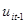and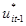are the estimated residuals from equation (3), which are the one-year lag of estimated residual and the one-year lag of spatial lag of estimated residual, respectively. Moreover,denotes the error correction term, anddenotes its spatially lagged counterpart. In Model 4, the estimate for the local error correction term,, is negative and statistically significant, indicating that regional production function is cointegrated. However, the estimate for the spatial error correction term,, is statistically significantly positive. It means that when the neighboring provincial GDP increases, local provincial GDP will increase in the next period as the error correction effect spillovers. This makes local provincial GDP to run high, so that local error correction makes them decrease. Furthermore, the estimates of the short-run term of most spatial lag variables are also positive and significant, suggesting that there are short-run spillover effects from the neighboring provincial provinces.

To compare with the estimated results without spatial correlation effect, we adopt the estimated residual of Model 1 to explain the long-run convergent effect. In Model 5, we find that the error-correction term is negative and significant and its value is in the interval between -1 and 0. This result implies a long-run convergence relationship. But all of these estimated values (absolute value) in Model 5 are larger than those in Model 4, implying the rate of convergence is slower than that when the spatial spillover effect is considered. The above results are somehow different from the findings in Liu et al. (2002), Narayan and Smyth (2004), and Kanbur and Zhang (2005), which give us a new insight on the issues of China’s regional economic convergence.

Table 3. Spatial error correction model estimation.

 Variables Model 4 (MLE) Coeff. (Std. err.) Model 5 (MLE) Coeff. (Std. err.)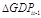0.3644***(0.0642) 0.5706*** (0.0285)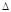CAPit-1 -0.0030(0.0064) -0.0120 (0.0082)LABit-1 -0.1425(0.1199) -0.2472* (0.1305)HUMit-1 -0.0792(0.0466) -0.0842*** (0.0276)FDI it-1 0.0114*** (0.0044) 0.0190*** (0.0055)GDP*it-1 0.0571 (0.0767)CAP*it-1 0.0826** (0.0343)LAB*it-1 0.5516** (0.3135)HUM*it-1 0.1930*** (0.0530)FDI*it-1 0.1946*** (0.0117) u it-1 -0.1338***(0.0315) -0.1518*** (0.0136) u* it-1 0.3992***(0.0947) intercept 0.0236**(0.0097) 0.1061*** (0.0094) Log likelihood 714.547 582.935 LR test 847.33*** 584.11*** # of obs. 540 540

Note: The dependent variable is the first difference of regional GDP in the current period, dGDPit. u it-1 and u* it-1 in Model 4 are calculated from the residual of Model 3, and u it-1 in Model 5 is measured from Model 1.

4. Conclusion

The empirical findings can be summarized as follows. First, the estimated results clearly indicate that a strong spatial dependence over the variables in China’s regional production function. Second, based on the presence of cross-sectional dependence in panel and given the variables among the regional production function have the same order of integrated, we evidence that the variables among the regional production function are cointegrated, which are tested using the methodologies of Kao (1999) and Pedroni (1999). Finally, by estimating a spatial error correction model, we find the local cointegration term is significantly negative, suggesting a long-run convergence relation for the Chinese regional economic growth.

References

1. Anselin, L. (1995), Local indicators of spatial association—LISA , Geographical analysis, 27 (2), 93-115.
2. Anselin, L. (1988), Spatial econometrics. Dordrecht, the Netherlands: Kluwer Academic.
3. Anselin, L. (2005), Exploring Spatial Data with GeoDaTM: A Workbook, Center for Spatially Integrated Social Science.
4. Baker, D., Merkert, R. and Kamruzzaman, M., (2015) Regional aviation and economic growth: cointegration and causality analysis in Australia, Journal of Transport Geography, 43, pp 140-150.
5. Baltagi B.H. and C. Kao (2000), Nonstationary Panels, Cointegration in Panels and Dynamic Panels: A Survey, in Badi Baltagi, Thomas B. Fomby and R. Carter Hill, editors, Vol. 15, 7-51.
6. Baltagi, B.H. (2005), Econometric Analysis of Panel Data, 3nd edition New York: Wiley.
7. Baltagi, B.H., G. Bresson, and A. Pirotte (2007), Panel Unit Root Tests and Spatial Dependence, Journal of Applied Econometrics 22, 339-360.
8. Basile, R., M. Costantini and S, Destefanis (2007), Unit Root and Cointegration Tests for Cross-Sectionally Correlated Panels-Estimating Regional Production Functions, Working Paper.
9. Beenstock, M. and D. Felsenstein (2007), Spatial Vector Autoregressions, Spatial Economic Analysis 2(2), 167-196.
10. Beenstock, M. and D. Felsenstein (2010), Spatial Error Correction and Cointegration in Nonstationary Panel Data: Regional House Prices in Israel, Journal of Geographical Systems, Vol. 12, No. 2, pp 189-206.
11. Breusch, T. and A. Pagan (1979), A Simple Test for Heteroskedasticity and Random Coefficient Variation, Econometrica 47, 1287-1294.
12. Chang, Y. (2002), Nonlinear IV Panel Unit Root Tests with Cross-Sectional Dependency, Journal of Econometrics 110, 261–292.
13. Chiang, Min–Hsien and Chihwa Kao (2002), Nonstationary panel time series using NPT 1.3 – A user guide. Center for Policy Research, Syracuse University.Choi, I. (2001), Unit Root Tests for Panel Data, Journal of International Money and Finance 20, 249–272.
14. Corbae D, Durlauf SN, Hansen B (eds.). Cambridge University Press: Cambridge, UK; 311–333.
15. Elhorst, J. P. (2001), Dynamic Models in Space and Time. Geographical Analysis 33, 119–140.
16. Elhorst, J. P. (2003). Specification and Estimation of Spatial Panel Data Models, International Regional Science Review 26. 244–268.
17. Elhorst, J. P. (2004), Serial and Spatial Dependence in Space–Time Models. In Spatial Econometrics and Spatial Statistics, edited by A. Getis, J. Mur, and H. G. Zoller. New York: Palgrave.
18. Elhorst, J. P. (2009), Spatial Panel Data Models. In Fischer MM, Getis A (Eds.) Handbook of Applied Spatial Analysis, Ch. C.2. Springer: Berlin Heidelberg New York.
19. Fingleton, B., (1999), Spurious Spatial Regression: Some Monte Carlo Results with a Spatial Unit and Spatial Cointegration, Journal of Regional Science 39(1), 1-19.
20. Geary, R., (1954), The contiguity ratio and statistical mapping, The IncorporatedStatistician5, 115–145.
21. Hadri, K. (2000), Testing for Unit Roots in Heterogeneous Panel Data, Econometrics Journal 3, 148-161.
22. Hsiao, C. (1986), Analysis of Panel Data, Cambridge, Cambridge University Press.
23. Hsiao, C., M. H. Pesaran, and A. K. Tahmiscioglu (2002), Maximum likelihood estimationof fixed effects dynamic panel data models covering short time periods. Journal of Econometrics 109, 107–150.
24. Im, K. S., Pesaran, M. H., and Y. Shin (2003), Testing for Unit Roots in Heterogeneous Panels, Journal of Econometrics 115, 53-74.
25. Kao, C. (1999), Spurious Regression and Residual–Based Tests for Cointegration in Panel Data, Journal of Econometrics 90 (1), 1–44.
26. Kao, C., M. Chiang, and B, Chen (1999), International R&D Spillovers: An Application of Estimation and Inference in Panel Cointegration, Oxford Bulletin of Economics and Statistics, 61, 691-709.
27. Kanbur, R. and X. Zhang(2005), Fifty Years of Regional Inequality in China: a Journey Through Central Planning, Reform, and Openness, Review of Development Economics 9(1), 87–106.
28. Kosfeld, Reinhold and Jorgen Lauridsen, (2004), "Dynamic spatial modeling of regional convergence processes,’ Empirical Economics 29, pp. 705-722.
29. Kuo, C.C. and C.H. Yang (2008), Knowledge Capital and Spillover on Regional Economic Growth: Evidence from China, China Economic Review 19, 594-604.
30. Lauridsen, J. and R. Kosfeld (2006), A Test Strategy for Spurious Spatial Regression, Spatial Nonstationairy, and Spatial Cointegration, Paper in Regional Science 85, 363-377.
31. Lauridsen, J. and R. Kosfeld (2007), Spatial Cointegration and Heteroscedasticity, Journal of Geography Systems 9, 253-265.
32. LeSage, J. P. (1999). The theory and practice of spatial econometrics, econometrics toolbox for MATLAB, available on the internet athttp://www.spatial-econometrics.com/.
33. Levin, A., C.F. Lin and C.S.J. Chu (2002), Unit Root Tests in Panel Data: Asymptotic and Finite-Sample Properties, Journal of Econometrics 108, 1-24.
34. Liao, F. H., Wei, Y. D. (2015), Space, scale, and regional inequality in provincial China: A spatial filtering approach, Applied Geography, 61, 94-104.
35. Liu, X., P. Burridge, and P. J. N. Sinclair (2002), Relationships between economic growth, foreign direct investment and trade: evidence from China, Applied Economics 34, 1433-1440.
36. Maasoumi, E. and L. Wang (2008), Economic reform, growth and convergence in China, Econometrics Journal 11, 128–154.
37. Maddala, G. and S. Wu (1999), A Comparative Study of Unit Root Tests and a New Simple Test, Oxford Bulletin of Economics and Statistics 61, 631-652.
38. Mankiw, N.G., D. Romer, and D. N. Weil (1992), A Contribution to The Empirics of Economic Growth, The Quarterly Journal of Economics, 407-437.
39. McCoskey, S. and C. Kao (1998), A Residual-based of the Null Hypothesis ofCointegration in Panel Data, Econometrics Reviews, 17, 57-84.
40. Moran, P. A. P. (1948). The interpretation of statistical maps. Biometrika 35. 255-260.
41. Narayan, P. K. and R. Smyth (2004), Temporal Causality and the Dynamics of Exports, Human Capital and Real Income in China, International Journal of Applied Economics 1(1), 24-45.
42. Newey, W. and K. West (1994), Autocovariance lag selection in covariance matrix estimation, Review of Economic Studies 61, 613–653.
43. Pedroni, P. (1999), Critical Values for Cointegration Tests in Heterogeneous Panels with Multiple Regressors, Oxford Bulletin of Economics and Statistics 61, 653-70.
44. Pedroni, P. and J. Y. Yao (2005), Regional income Divergence in China, working paper.
45. Pesaran, M.H. (2004), General diagnostic tests for cross section dependence in panels. CESifo Working Papers No.1233.
46. Pesaran, M. H. (2007), A Simple Panel Unit Root Test in The Presence of Cross- Section Dependence. Journal of Applied Econometrics 22, 265–312.
47. Solow, R.M. (1957), Technical Change and the Aggregate Production Function, Review of Economics and Statistics, 9, 312-320.
48. Xu, L. C. and H.-F. Zou (2000), Explaining the changes of income distribution in china. China Economic Review 11, 149–70.
49. Yao, S. (2006), On Economic Growth, FDI and Exports in China, Applied Economics 38, 339-351.
50. Zhou, Y., Li, N., Wu, W., Wu, J., (2014), Evolution of spatial-temporal pattern of county economic development in China during 1982-2010, Progress in Geography, 33(1), 102-113.
51. Zou ,W., Zhuang, Z., Zhou, H. and Song, H. (2008), Measuring divergence in provincial growth in China: 1981–2004, Journal of Economic Policy Reform 11(3), 215–227.

 Contents 1. 2. 2.1. 2.2. 2.3. 2.4. 2.5. 3. 4.
Article ToolsAbstractPDF(241K)